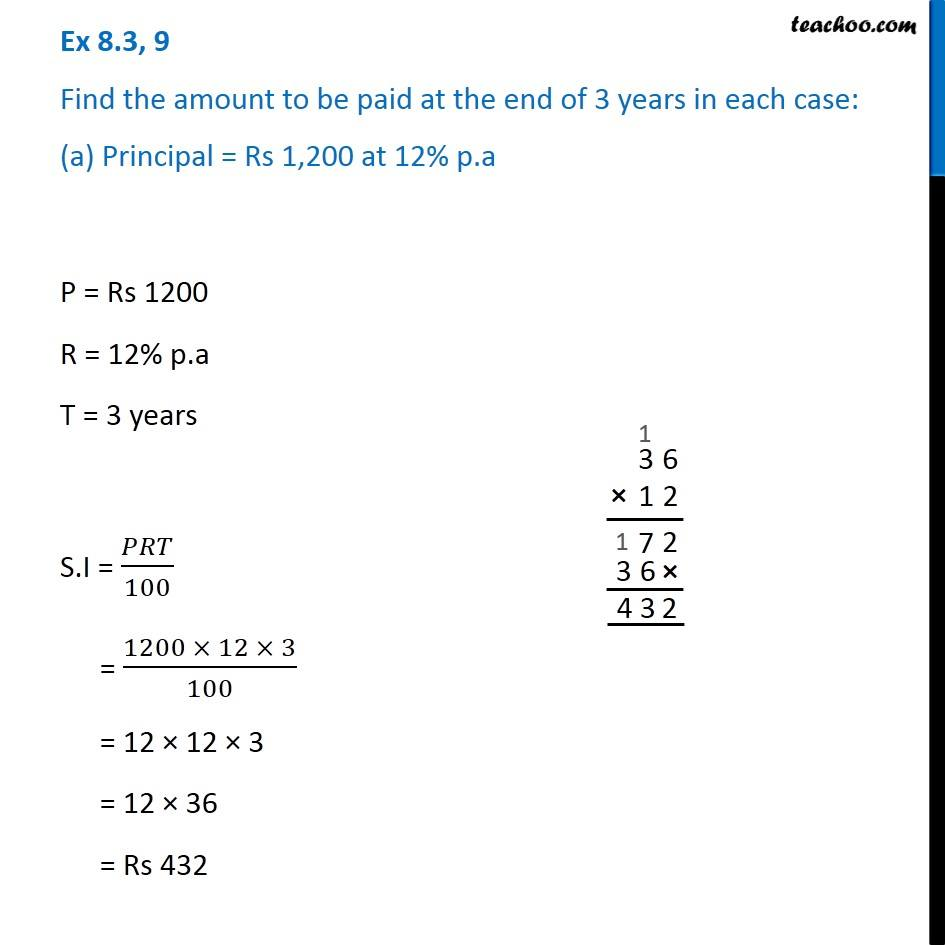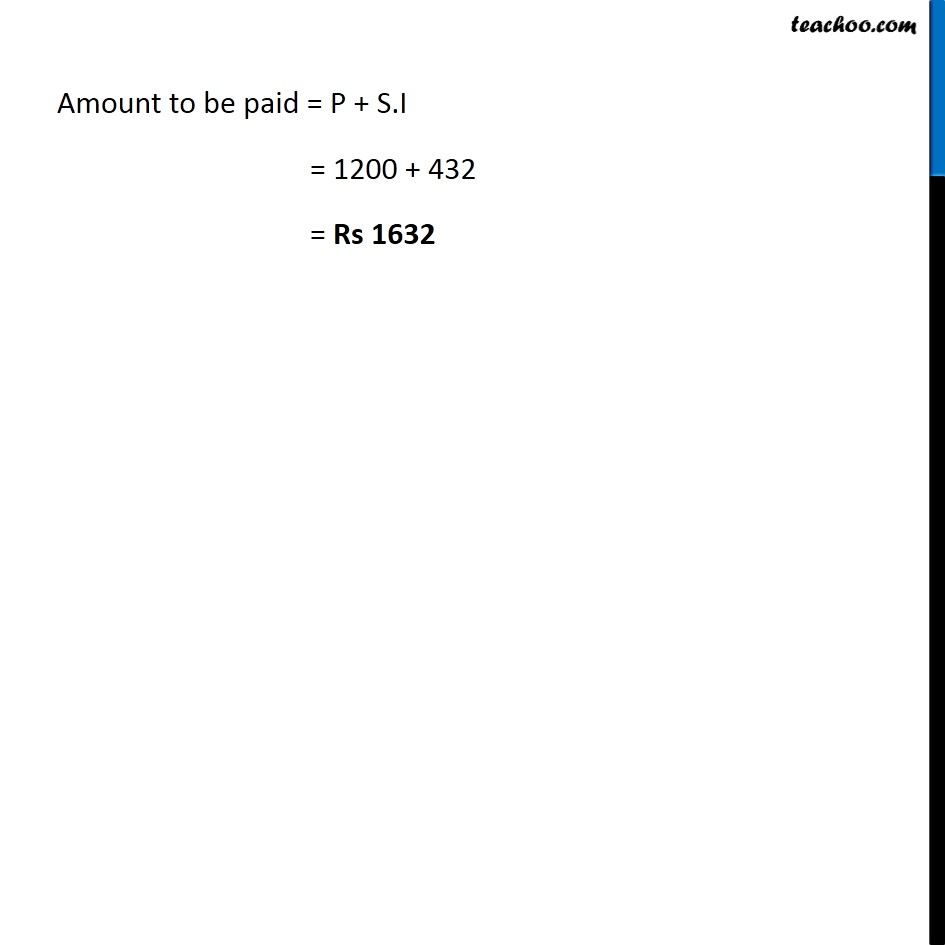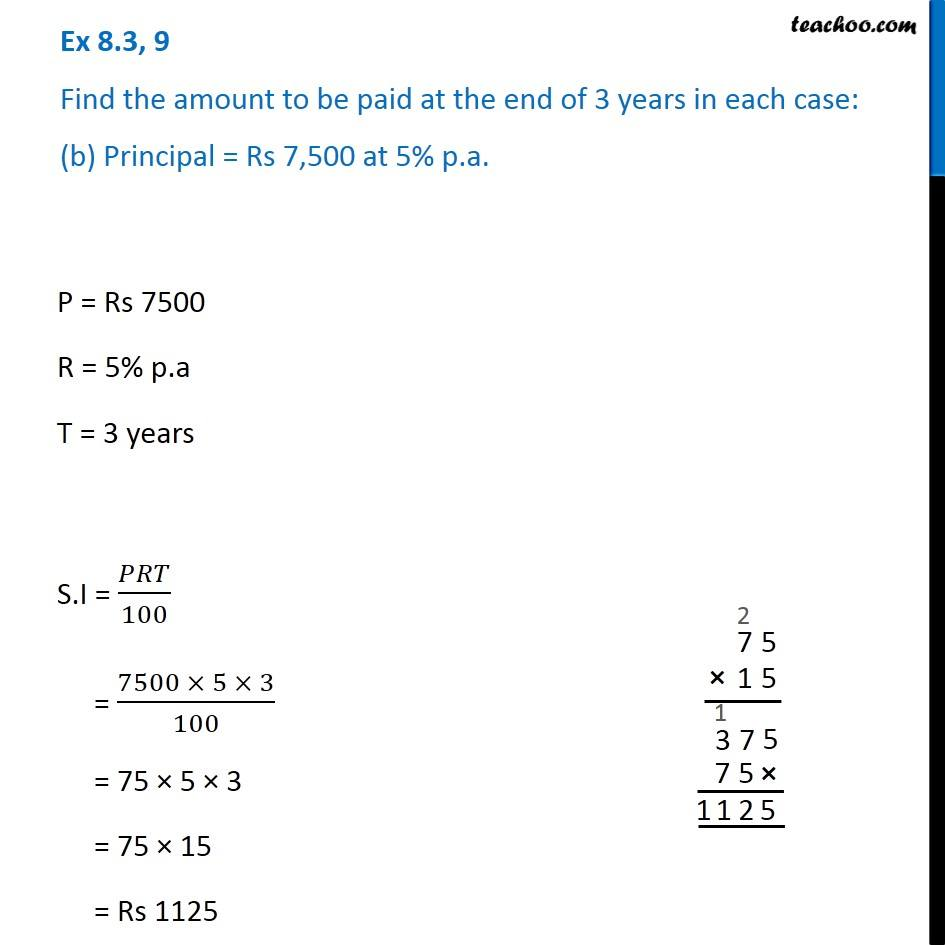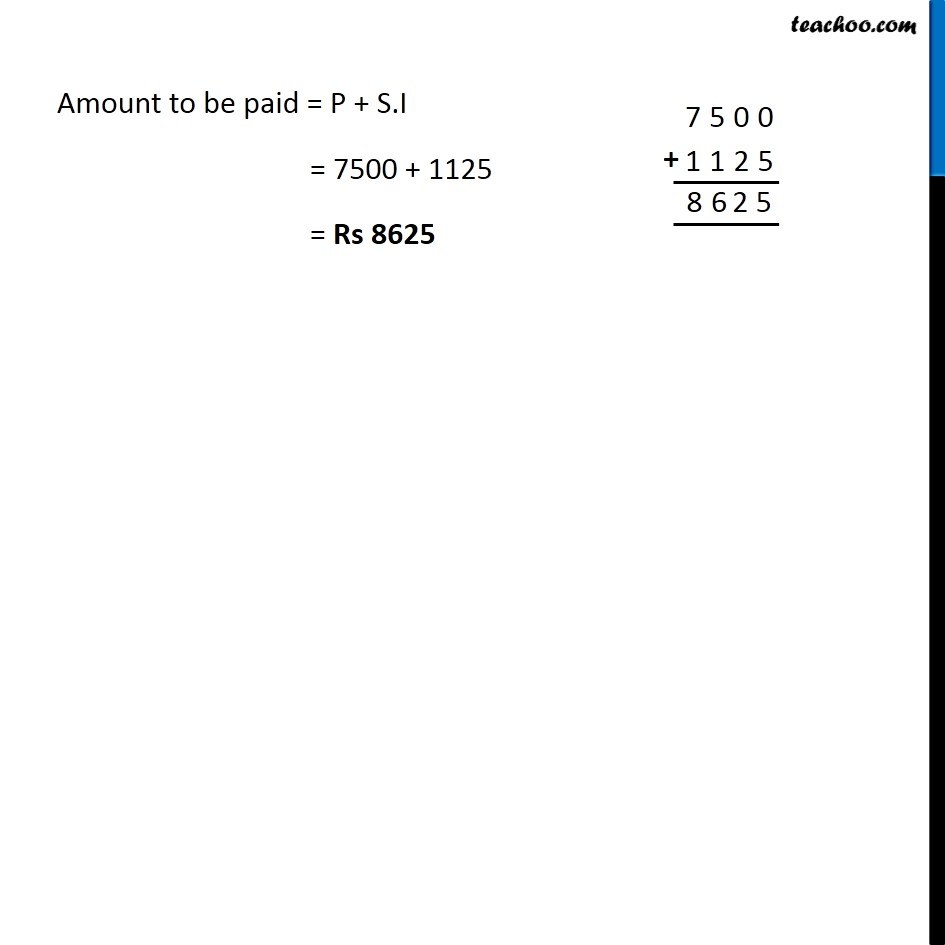Subscribe to our Youtube Channel - https://you.tube/teachoo

1. Chapter 8 Class 7 Comparing Quantities
2. Serial order wise
3. Ex 8.3

Transcript

Ex 8.3, 9 Find the amount to be paid at the end of 3 years in each case: (a) Principal = Rs 1,200 at 12% p.a P = Rs 1200 R = 12% p.a T = 3 years S.I = 𝑃𝑅𝑇/100 = (1200 × 12 × 3)/100 = 12 × 12 × 3 = 12 × 36 = Rs 432 Amount to be paid = P + S.I = 1200 + 432 = Rs 1632 Ex 8.3, 9 Find the amount to be paid at the end of 3 years in each case: (b) Principal = Rs 7,500 at 5% p.a. P = Rs 7500 R = 5% p.a T = 3 years S.I = 𝑃𝑅𝑇/100 = (7500 × 5 × 3)/100 = 75 × 5 × 3 = 75 × 15 = Rs 1125 Amount to be paid = P + S.I = 7500 + 1125 = Rs 8625

Ex 8.3